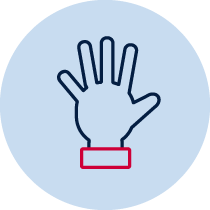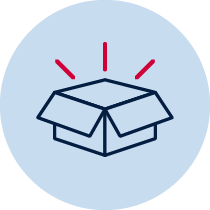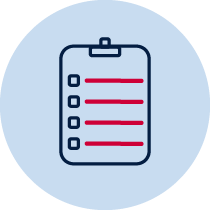# Area – Which shape has the larger area?

Students compare the area of two shapes that cannot be transposed, use uniform informal units to measure with no gaps or overlaps, and iterate a single informal unit and use the row and column structure to measure area.Practical Resource required Take photo Teacher observation Individual

## Measurement and geometry – area

• describes mathematical situations and methods using everyday and some mathematical language, actions, materials, diagrams and symbols MA1-1WM
• measures, records, compares and estimates areas using uniform informal units MA1-10MG

## Content

• Measure and compare areas using uniform informal units

MA1-6NA – uses a range of mental strategies and concrete materials for multiplication and division

## National Numeracy Learning Progression mapping to the NSW mathematics syllabus

When working towards the outcome MA1-10MG the sub-elements (and levels) of Understanding units of measurement (UuM3-UuM5) describe observable behaviours that can aid teachers in making evidence-based decisions about student development and future learning.

## Materials

• selection of units for measuring including squares from pattern blocks set, as well as other non-uniform materials and those that leave gaps such as round counters
• pencil
• coloured paper squares could be provided for students to paste on to the worksheet
• Which shape has the larger area? (DOCX 74.6KB)
• Teacher instructions

The purpose of this task is to gauge students’ understanding of area concepts such as:

• comparing the area of two shapes that cannot be transposed
• using uniform informal units to measure with no gaps or overlaps
• iterating a single informal unit to measure area
• using the row and column structure to measure area

Provide the student with the 2 shapes on two separate pieces of paper, so they cannot be superimposed but can be compared visually. Ask the student to estimate which shape they believe will be larger and why? Note the student’s reasoning.

Offer the student a selection of appropriate units, such as squares, and inappropriate units such as counters. Can they select one unit and use this to help them prove they are correct? If the student is unsure, provide the student with a collection of materials and ask them to measure the shapes to check their answer. Observe the student as they are measuring to see how they are using the materials and how they count to determine the total (by ones, skip counting, or multiplication).

Ask the student to identify which shape was larger and reflect on their original prediction, as well as the units they chose to measure the shapes.

## Student instructions

• Estimate which shape is larger in area. Circle the name of the shape that you estimate is larger.
• Measure the area of shapes?
• Which shape is larger in area? Explain how you know.

## Possible areas for further exploration

• Attempting to iterate or use row and column structure but drawings are 'crooked', resulting in inaccurate representation.
• Using inappropriate units, such as counters, which leave gaps.
• Not attending to the baseline when placing units on the shape which leads to inaccurate measurement.

## Where to next?

For students who are able to use one unit to iterate to measure, they may be ready for the introduction of formal units of measurement for area.

Students may also be extended by using different shapes, including composite rectangles and squares, where the row and column structure can still be used, or shapes without right angles, such as triangles or trapeziums, so students can consider how to approach partial units.